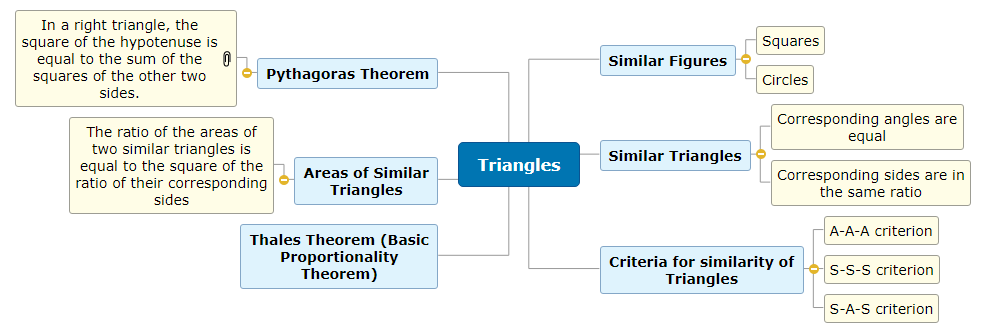Start Mind Mapping Now!
• Professional Mind Mapping
• Best MS Office Integration
• Build-in Gantt chart & Timeline
• Templates & Tutorials

# Triangles - Mind Map## Triangles - Mind Map

46 days ago by: Shambhu Singh

Mind Map of Triangles for Class X
• Triangles
• Similar Figures
• Squares
• Circles
• Similar Triangles
• Corresponding angles are equal
• Corresponding sides are in the same ratio
• Criteria for similarity of Triangles
• A-A-A criterion
• S-S-S criterion
• S-A-S criterion
• Thales Theorem (Basic Proportionality Theorem)
• Areas of Similar Triangles
• The ratio of the areas of two similar triangles is equal to the square of the ratio of their corresponding sides
• Pythagoras Theorem
• In a right triangle, the square of the hypotenuse is equal to the sum of the squares of the other two sides.
Report abuse
More Maps by This User